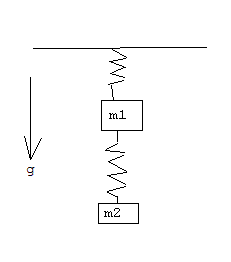# small oscillations question

## Recommended Posts

Hi

see the attached picture...

2 coupled masses, each suspended from spring in gravitational field...

also entire construction can vibrate only vertically...

I need to write lagrangian for this system in the following form:

$L = \frac{1}{2}\sum_{i=1,j=1}^{2}m_{ij}\dot{x}_i\dot{x}_j-\frac{1}{2}\sum_{i=1,j=1}^{2}k_{ij}x_ix_j$

$x_i$ and $x_j$ are displacements of masses from equalibrium positions and springs are identical

the kinetic part of L is easy one but i stack with potential part...

i get to this expression(i always confused about right signs):

$m_1g(l+x_1)+m_2g(l+x_1+l+x_2) + \frac{1}{2}m_1{x_1}^2+\frac{1}{2}m_2{x_2}^2$

- positive direction taken along g direction

- l is length of unstreched spring

every potential term in L must in $k_{ij}x_ix_j$ form but i don't see a way how do i get it from my expression for potential energy because there are g force terms containing only $x_i$ or $x_j$

thanks##### Share on other sites

Ok, I tried to calculate de Lagrangian and I arrived to this expression:

L = T + m1·g·x1 + m2·g·x2 +1/2·k·[2·x1^2 + x2^2 - 2·x1·x2]

where T is the same kinetic term you wrote, and the other terms are minus the potential energy (-U).

I calculate this supposing that the origin of coordinates is located at the roof and is positive downwards. I called x1, and x2 the positions of the masses from the origin.

For the first mass you have: U1 = - m1·g·x1 - 1/2·k(x1 - l)^2.

And for the second mass: U2 = - m2·g·x2 - 1/2·k·(x2 - x1 - l)^2.

Expanding these terms and just keeping terms of the form xi·xj you arrive to the expression I wrote above.

Observe that there is a term that couples both masses.

I am sorry, i don't know how to insert a formula as an image.

Hope this helps.

Greetings.

Nicolas

##### Share on other sites

Corrected version (using LaTeX) of my previous post.

$L=T+m_1gx_1+m_2gx_2+\frac{k}{2}(2x_1^2+x_2^2-2x_1x_2)$

where $T$ is the same kinetic term you wrote, and the other terms are minus the potential energy $(-U)$.

I calculate this supposing that the origin of coordinates is located at the roof and is positive downwards. I called $x_1$, and $x_2$ the positions of the masses from the origin.

For the first mass you have: $U_1=-m_1gx_1-\frac{k}{2}(x_1-l)^2$.

And for the second mass: $U_2=-m_2gx_2-\frac{k}{2}(x_2-x_1-l)^2$.

Expanding these terms and just keeping terms of the form $x_ix_j$ you arrive to the expression I wrote above.

Observe that there is a term that couples both masses.

Greetings.

Nicolas

## Create an account

Register a new account